# 机器学习-吴恩达：学习笔记及总结（10）

## Coursera | Machine Learning | Andrew Ng

Thistledown    June 25, 2020

## 34 Gradient Descent with Large Datasets

#### 34.1 Learning With Large Datasets

• If you look back at 5-10 year history of machine learning, ML is much better now because we have much more data

• However, with this increase in data comes great responsibility? No, comes a much more significant computational cost
• New and exciting problems are emerging that need to be dealt with on both the algorithmic and architectural level
• One of best ways to get high performance is take a low bias algorithm and train it on a lot of data

• But learning with large datasets comes with its own computational problems
• Because of the computational cost of this massive summation, we’ll look at more efficient ways around this

• Either using a different approach
• Optimizing to avoid the summation
• First thing to do is ask if we can train on 1000 examples instead of 100,000,000

• Randomly pick a small selection
• Can you develop a system which performs as well?
• Sometimes yes - if this is the case you can avoid a lot of the headaches associated with big data
• To see if taking a smaller sample works, you can sanity check by plotting error vs. training set size

• If our plot looked like this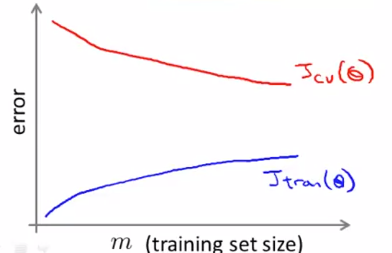• Looks like a high variance problem

• More examples should improve performance
• If plot looked like this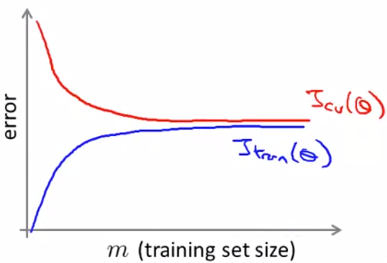• This looks like a high bias problem

• More examples may not actually help - save a lot of time and effort if we know this before hand
• One natural thing to do here might be to:
• Add extra hidden units (if using neural networks)

• For many learning algorithms, we derived them by coming up with an optimization objective (cost function) and using an algorithm to minimize that cost function

• When you have a large dataset, gradient descent becomes very expensive
• So here we’ll define a different way to optimize for large data sets which will allow us to scale the algorithms
• Suppose you’re training a linear regression model with gradient descent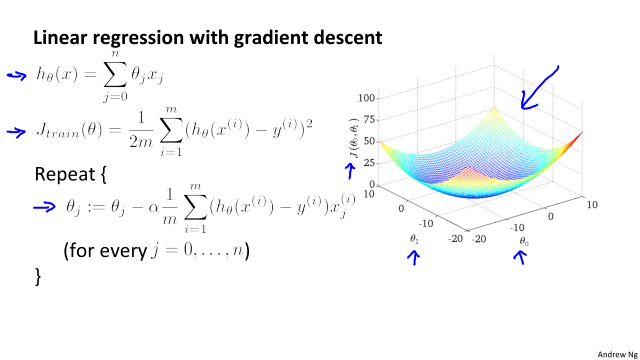• We will use linear regression for our algorithmic example here when talking about stochastic gradient descent, although the ideas apply to other algorithms too, such as

• Logistic regression
• Neural networks
• Below we have a contour plot for gradient descent showing iteration to a global minimum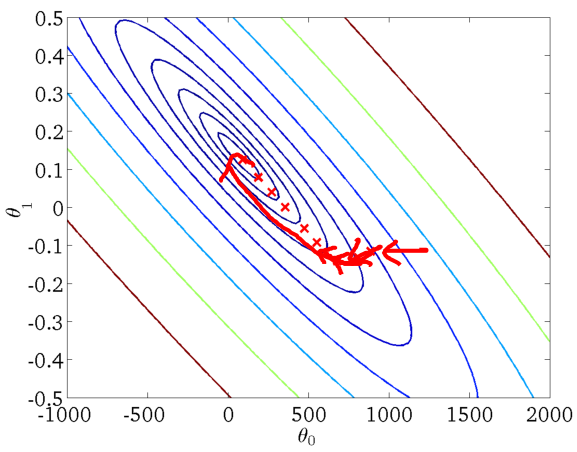• As mentioned, if $m$ is large gradient descent can be very expensive

• Although so far we just referred to it as gradient descent, this kind of gradient descent is called batch gradient descent

• This just means we look at all the examples at the same time
• Batch gradient descent is not great for huge datasets

• If you have 300,000,000 records you need to read in all the records into memory from disk because you can’t store them all in memory
• By reading all the records, you can move one step (iteration) through the algorithm
• Then repeat for EVERY step
• This means it take a LONG time to converge
• Especially because disk I/O is typically a system bottleneck anyway, and this will inevitably require a huge number of reads
• What we’re going to do here is come up with a different algorithm which only needs to look at single example at a time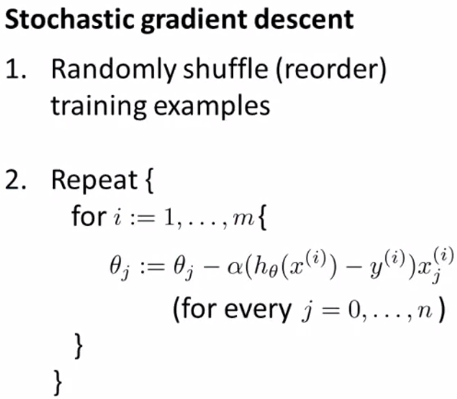• Define our cost function slightly differently, as $\operatorname{cost}\left(\theta,\left(x^{(i)}, y^{(i)}\right)\right)=\frac{1}{2}\left(h_{\theta}\left(x^{(i)}\right)-y^{(i)}\right)^{2}$
• So the function represents the cost of $\theta$ with respect to a specific example $(x^{(i)}, y^{(i)})$
• And we calculate this value as one half times the squared error on that example
• Measures how well the hypothesis works on a single example
• The overall cost function can now be re-written in the following form: $J_{t r a i n}(\theta)=\frac{1}{m} \sum_{i=1}^{m} \operatorname{cost}\left(\theta,\left(x^{(i)}, y^{(i)}\right)\right)$
• This is equivalent to the batch gradient descent cost function
• With this slightly modified (but equivalent) view of linear regression we can write out how stochastic gradient descent works
• So what’s going on here?
• The term $(h_\theta(x^{(i)}) - y^{(i)})x_j^{(i)}$
• Is the same as that found in the summation for batch gradient descent
• It’s possible to show that this term is equal to the partial derivative with respect to the parameter $θ_j$ of the $\operatorname{cost}\left(\theta,\left(x^{(i)}, y^{(i)}\right)\right)$
• What stochastic gradient descent algorithm is doing is scanning through each example
• The inner for loop does something like this…
• Looking at example 1, take a step with respect to the cost of just the 1st training example
• Having done this, we go on to the second training example
• Now take a second step in parameter space to try and fit the second training example better
• Now move onto the third training example
• And so on…
• Until it gets to the end of the data
• We may now repeat this who procedure and take multiple passes over the data
• The randomly shuffling at the start means we ensure the data is in a random order so we don’t bias the movement
• Randomization should speed up convergence a little bit
• Although stochastic gradient descent is a lot like batch gradient descent, rather than waiting to sum up the gradient terms over all $m$ examples, we take just one example and make progress in improving the parameters already
• Means we update the parameters on EVERY step through data, instead of at the end of each loop through all the data
• What does the algorithm do to the parameters?

• As we saw, batch gradient descent does something like this to get to a global minimum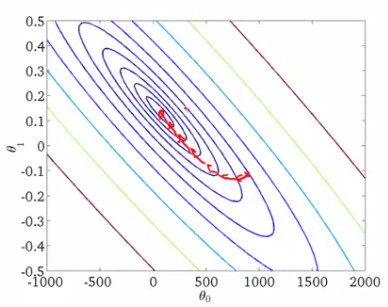• With stochastic gradient descent every iteration is much faster, but every iteration is flitting a single example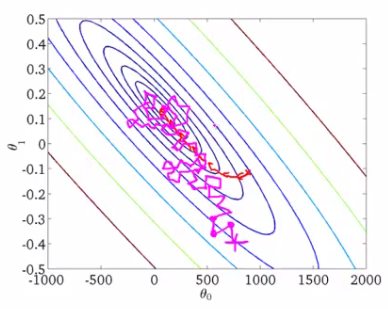• What you find is that you “generally” move in the direction of the global minimum, but not always
• Never actually converges like batch gradient descent does, but ends up wandering around some region close to the global minimum
• In practice, this isn’t a problem - as long as you’re close to the minimum that’s probably OK
• One final implementation note

• May need to loop over the entire dataset 1-10 times
• If you have a truly massive dataset it’s possible that by the time you’ve taken a single pass through the dataset you may already have a perfectly good hypothesis
• In which case the inner loop might only need to happen 1 if $m$ is very very large
• If we contrast this to batch gradient descent

• We have to make $k$ passes through the entire dataset, where $k$ is the number of steps needed to move through the data

• Mini-batch gradient descent is an additional approach which can work even faster than stochastic gradient descent

• To summarize our approaches so far

• Batch gradient descent: Use all $m$ examples in each iteration
• Stochastic gradient descent: Use 1 example in each iteration
• Mini-batch gradient descent: Use $b$ examples in each iteration
• $b$ = mini-batch size (typical range for $b$ = 2-100 (10 maybe))
• For example

• $b$ = 10
• Get 10 examples from training set
• Perform gradient descent update using the ten examples
• Mini-batch algorithm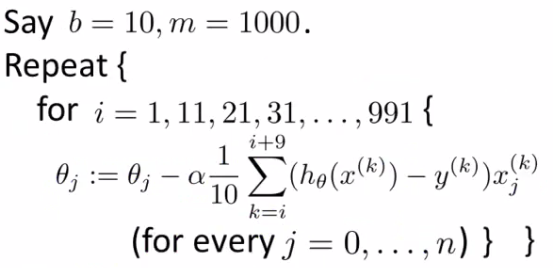• We for-loop through $b$-size batches of $m$
• Compared to batch gradient descent this allows us to get through data in a much more efficient way
• After just $b$ examples we begin to improve our parameters
• Don’t have to update parameters after every example, and don’t have to wait until you cycled through all the data

• Why should we use mini-batch?
• Allows you to have a vectorized implementation
• Means implementation is much more efficient
• Can partially parallelize your computation (i.e. do 10 at once)
• A disadvantage of mini-batch gradient descent is the optimization of the parameter $b$
• But this is often worth it!
• To be honest, stochastic gradient descent and batch gradient descent are just specific forms of batch-gradient descent
• For mini-batch gradient descent, $b$ is somewhere in between 1 and $m$ and you can try to optimize for it

#### 34.4 Stochastic Gradient Descent Convergence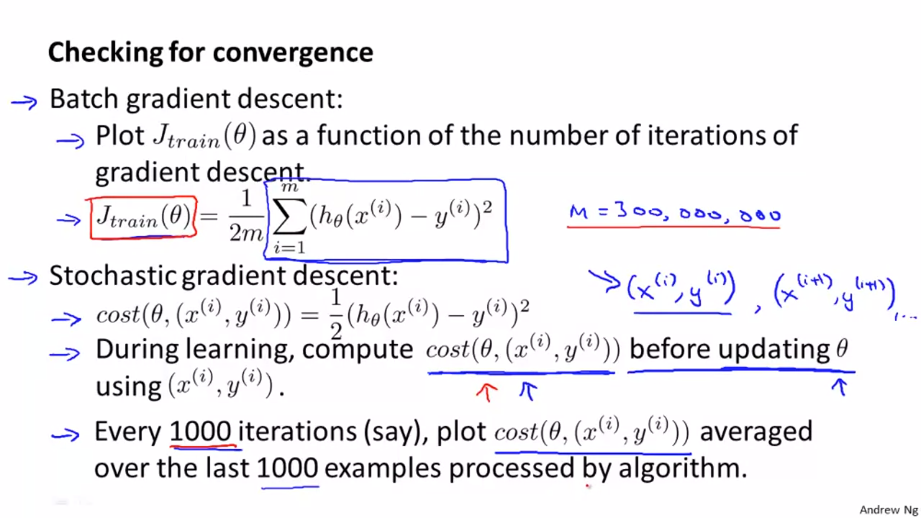• With batch gradient descent, we could plot cost function vs number of iterations
• Should decrease on every iteration
• This works when the training set size was small because we could sum over all examples
• Doesn’t work when you have a massive dataset
• We don’t want to have to pause the algorithm periodically to do a summation over all data
• Moreover, the whole point of stochastic gradient descent is to avoid those whole-data summations
• For stochastic gradient descent, we have to do something different
• Take cost function definition $\operatorname{cost}\left(\theta,\left(x^{(i)}, y^{(i)}\right)\right)=\frac{1}{2}\left(h_{\theta}\left(x^{(i)}\right)-y^{(i)}\right)^{2}$
• One half the squared error on a single example
• While the algorithm is looking at the example $\left(x^{(i)}, y^{(i)}\right)$, but before it has updated $\theta$ we can compute the cost of the example $cost\left(x^{(i)}, y^{(i)}\right)$
• i.e. we compute how well the hypothesis is working on the training example
• Need to do this before we update $\theta$ because if we did it after $\theta$ was updated the algorithm would be performing a bit better (because we’d have just used $\left(x^{(i)}, y^{(i)}\right)$ to improve $\theta$)
• To check for the convergence, every 1000 iterations we can plot the costs averaged over the last 1000 examples
• Gives a running estimate of how well we’ve done on the last 1000 estimates
• By looking at the plots we should be able to check convergence is happening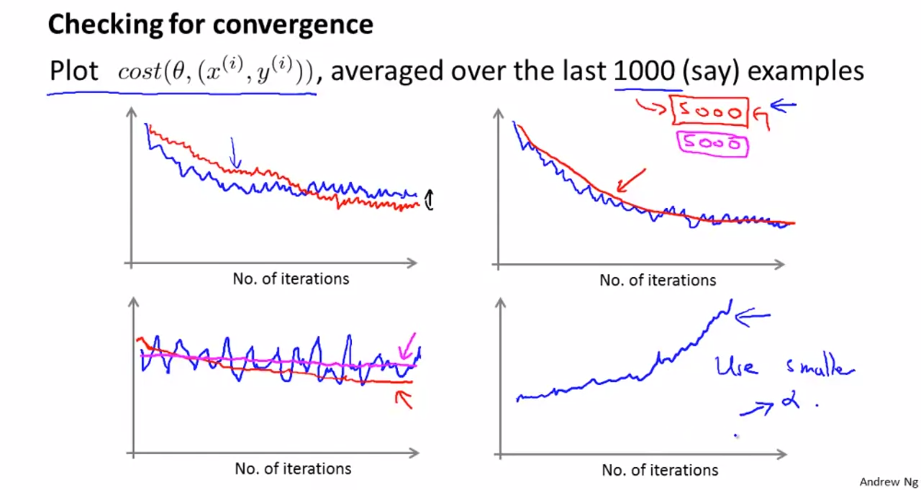• In general

• Might be a bit noisy (1000 examples isn’t that much)
• If you get a figure like this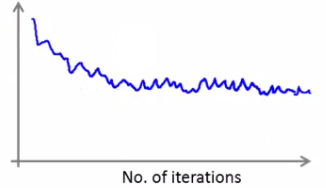• That’s a pretty decent run
• Algorithm may have convergence
• If you use a smaller learning rate you may get an even better final solution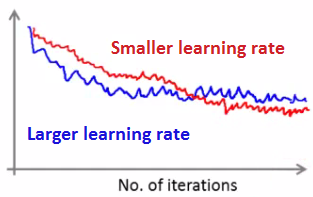• This is because the parameter oscillate around the global minimum
• A smaller learning rate means smaller oscillations
• If you average over 1000 examples and 5000 examples you may get a smoother curve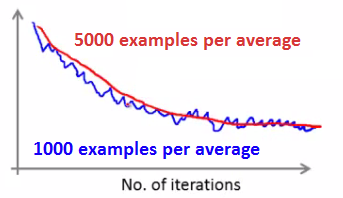• The disadvantage of a larger average means you get less frequent feedback
• Sometimes you may get a plot that looks like this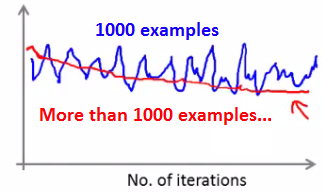• Looks like cost is not decreasing at all
• But if you then increase to averaging over a larger number of examples you do see this general trend
• Means the blue line was too noisy, and that noise is ironed out by taking a greater number of entries per average
• Of course, it may not decrease, even with a large number
• If you see a curve the looks like its increasing then the algorithm may be displaying divergence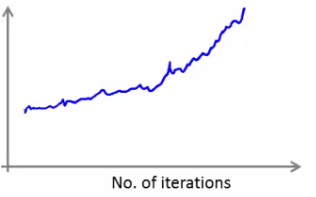• Should use a smaller learning rate
• Learning rate $\alpha$ is typically held constant. Can slowly decrease over time if we want $\theta$ to converge. (E.g. $\alpha = \frac{\text{const1}}{\text{iterationNumber}+\text{const2}}$)

#### 35.1 Online Learning

• New setting

• Allows us to model problems where you have a continuous stream of data you want an algorithm to learn from
• Similar idea of stochastic gradient descent, in that you do slow updates
• Web companies use various types of online learning algorithms to learn from traffic
• Can (for example) learn about user preferences and hence optimize your website
• Example - Shipping service

• Users come and tell you origin and destination
• You offer to ship the package for some amount of money ($10 -$50)
• Based on the price you offer, sometimes the user uses your service ($y = 1$), sometimes they don’t ($y = 0$)
• Build an algorithm to optimize what price we offer to the users
• Capture
• Origin and destination
• Work out
• What the probability of a user selecting the service is
• We want to optimize the price
• To model this probability we have something like
•  $p(y=1 x;\theta)$
• Probability that $y =1$, given $x$, parameterized by $\theta$
• Build this model with something like
• Logistic regression
• Neural network
• If you have a website that runs continuously an online learning algorithm would do something like this
• User comes - is represented as an $(x,y)$ pair where
• $x$ - feature vector including price we offer, origin, destination
• $y$ - if they chose to use our service or not
• The algorithm updates $\theta$ using just the $(x,y)$ pair: $\theta_j := \theta_j - \alpha \left(h_{\theta}(x) - y \right)x_j \quad (j=0, \dots, n)$
• So we basically update all the $\theta$ parameters every time we get some new data
• While in previous examples we might have described the data example as $(x^{(i)}, y^{(i)})$ for an online learning problem we discard this idea of a data “set” - instead we have a continuous stream of data so indexing is largely irrelevant as you’re not storing the data (although presumably you could store it)
• If you have a major website where you have a massive stream of data then this kind of algorithm is pretty reasonable

• You’re free of the need to deal with all your training data
• If you had a small number of users you could save their data and then run a normal algorithm on a dataset

• An online algorithm can adapt to changing user preferences

• So over time users may become more price sensitive
• The algorithm adapts and learns to this
• So your system is dynamic
• Another example - product search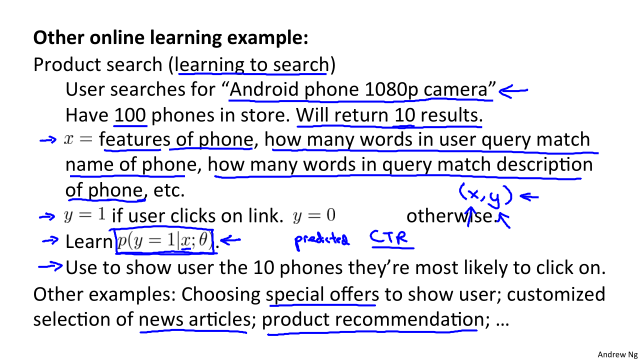• Other things you can do

• Special offers to show the user
• Show news articles - learn what users like
• Product recommendation
• These problems could have been formulated using standard techniques, but they are the kinds of problems where you have so much data that this is a better way to do things

#### 35.2 Map Reduce and Data Parallelism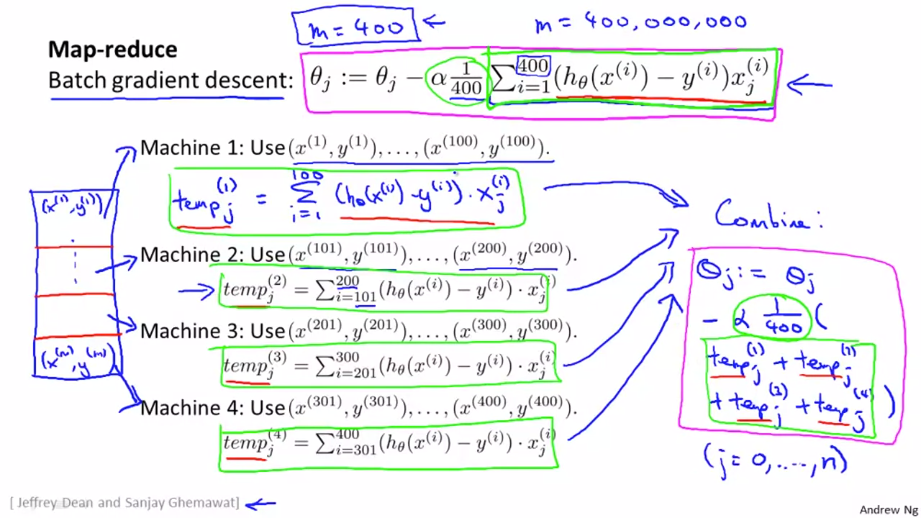• More generally map reduce uses the following scheme (e.g. where you split into 4)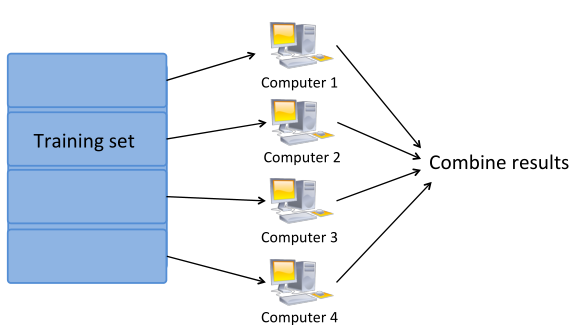• The bulk of the work in gradient descent is the summation

• Now, because each of the computers does a quarter of the work at the same time, you get a $4\times$ speedup
• Of course, in practice, because of network latency, combining results, it’s slightly less than $4\times$, but still good!
• Important thing to ask is: “Can algorithm be expressed as computing sums of functions of the training set?”

• Many algorithms can
• More broadly, by taking algorithms which compute sums you can scale them to very large datasets through parallelization

• Parallelization can come from
• Multiple machines
• Multiple CPUs
• Multiple cores in each CPU
• So even on a single compute can implement parallelization
• The advantage of thinking about Map Reduce here is because you don’t need to worry about network issues

• It’s all internal to the same machine
• Finally caveat/thought

• Depending on implementation detail, certain numerical linear algebra libraries can automatically parallelize your calculations across multiple cores
• So, if this is the case and you have a good vectorization implementation you can not worry about local Parallelization and the local libraries sort optimization out for you

## 36 Photo OCR

#### 36.1 Problem Description and Pipeline

• Case study focused around Photo OCR

• Three reasons to do this

• 1) Look at how a complex system can be put together
• 2) The idea of a machine learning pipeline
• What to do next
• How to do it
• 3) Some more interesting ideas
• Applying machine learning to tangible problems
• Artificial data synthesis
• What is the photo OCR problem?

• Photo OCR = Photo Optical Character Recognition
• With growth of digital photography, lots of digital pictures
• One idea which has interested many people is getting computers to understand those photos
• The photo OCR problem is getting computers to read text in an image
• Possible applications for this would include
• Make searching easier (e.g. searching for photos based on words in them)
• OCR of documents is a comparatively easy problem
• From photos it’s really hard
• OCR pipeline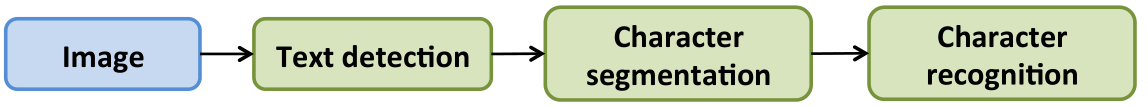• 1) Look through image and find text
• 2) Do character segmentation
• 3) Do character classification
• 4) some may do spell check after this too (Optional)
• We’re not focusing on such systems though
• Pipelines are common in machine learning

• Separate modules which may each be a machine learning component or data processing component
• If you’re designing a machine learning system, pipeline design is one of the most important questions

• Performance of pipeline and each module often has a big impact on the overall performance a problem
• You would often have different engineers working on each module
• Offers a natural way to divide up the workload

#### 36.2 Sliding Windows

• As mentioned, stage 1 is text detection

• Unusual problem in computer vision - different rectangles (which surround text) may have different aspect ratios (aspect ratio being height : width)
• Text may be short (few words) or long (many words)
• Tall or short font
• Text might be straight on
• Slanted
• Pedestrian detection

• Want to take an image and find pedestrians in the image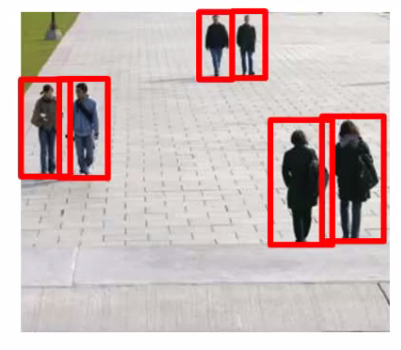• This is a slightly simpler problem because the aspect ration remains pretty constant

• Building our detection system

• Have $82 \times 36$ aspect ratio

• This is a typical aspect ratio for a standing human
• Collect training set of positive and negative examples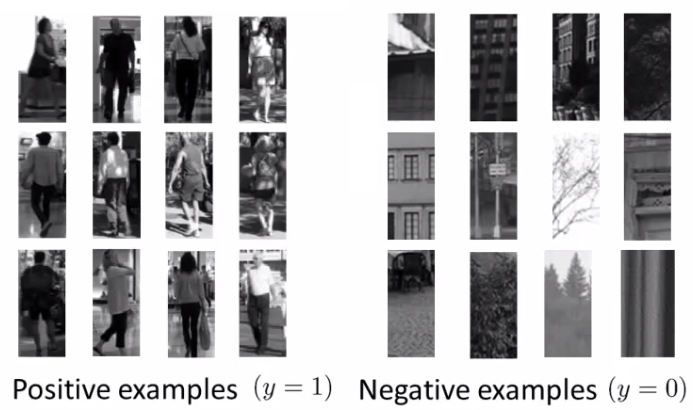• Could have 1000 - 10 000 training examples

• Train a neural network to take an image and classify that image as pedestrian or not

• Gives you a way to train your system
• Now we have a new image - how do we find pedestrians in it?

• Start by taking a rectangular $82 \times 36$ patch in the image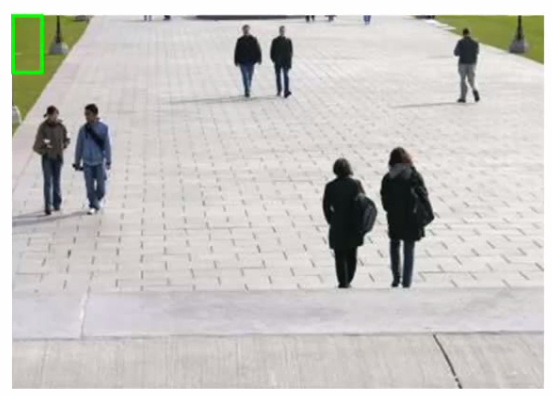• Run patch through classifier - hopefully in this example it will return $y = 0$
• Next slide the rectangle over to the right a little bit and re-run

• Then slide again
• The amount you slide each rectangle over is a parameter called the step-size or stride
• Could use 1 pixel
• Best, but computationally expensive
• More commonly 5-8 pixels used
• So, keep stepping rectangle along all the way to the right
• Eventually get to the end
• Then move back to the left hand side but step down a bit too
• Repeat until you’ve covered the whole image
• Now, we initially started with quite a small rectangle

• So now we can take a larger image patch (of the same aspect ratio)
• Each time we process the image patch, we’re resizing the larger patch to a smaller image, then running that smaller image through the classifier
• Hopefully, by changing the patch size and rastering repeatedly across the image, you eventually recognize all the pedestrians in the picture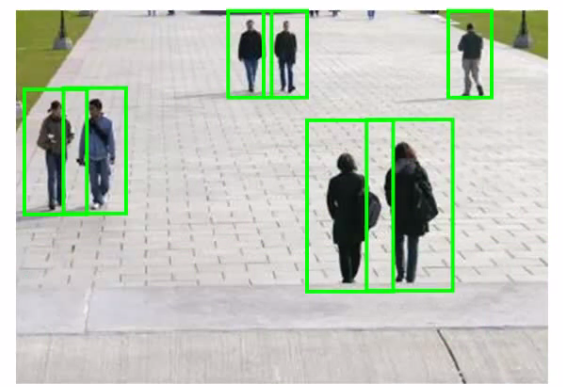• Text detection example

• Like pedestrian detection, we generate a labeled training set with

• Positive examples (some kind of text)
• Negative examples (not text)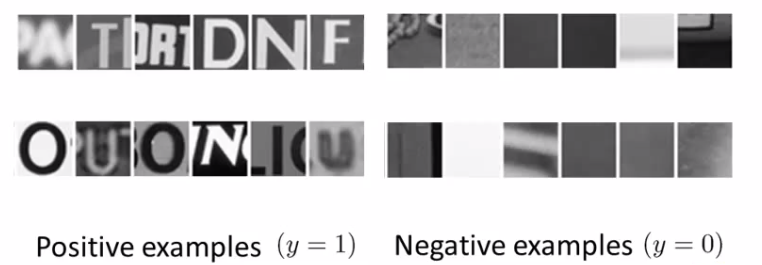• Having trained the classifier we apply it to an image

• So, run a sliding window classifier at a fixed rectangle size
• If you do that end up with something like this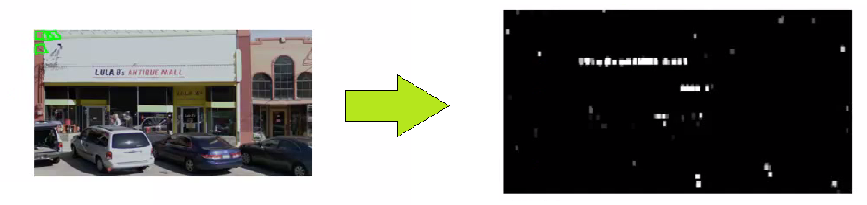• White region show where text detection system thinks text is

• Different shades of gray correspond to probability associated with how sure the classifier is the section contains text
• Black - no text
• White - text
• For text detection, we want to draw rectangles around all the regions where there is text in the image
• Take classifier output and apply an expansion algorithm

• Takes each of white regions and expands it

• How do we implement this

• Say, for every pixel, is it within some distance of a white pixel?
• If yes then color it white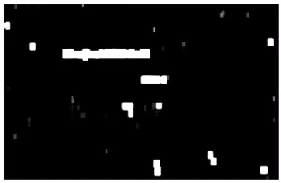• Look at connected white regions in the image above

• Draw rectangles around those which make sense as text (i.e. tall thin boxes don’t make sense)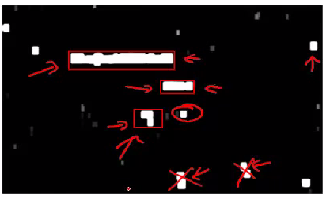• This example misses a piece of text on the door because the aspect ratio is wrong

• Stage 2: character segmentation

• Use supervised learning algorithm

• Look in a defined image patch and decide, is there a split between two characters?

• So, for example, our first training data item below looks like there is such a split
• Similarly, the negative examples are either empty or hold a full characters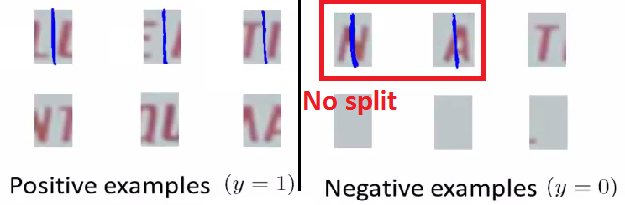• We train a classifier to try and classify between positive and negative examples

• Run that classifier on the regions detected as containing text in the previous section
• Use a 1-dimensional sliding window to move along text regions

• Does each window snapshot look like the split between two characters?
• If yes insert a split
• If not move on
• So we have something that looks like this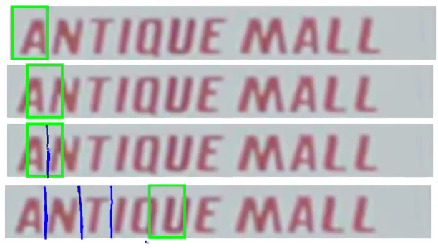• Character classification

• Standard OCR, where you apply standard supervised learning which takes an input and identify which character we decide it is
• Multi-class characterization problem

#### 36.3 Getting Lots of Data and Artificial Data

• We’ve seen over and over that one of the most reliable ways to get a high performance machine learning system is to take a low bias algorithm and train on a massive data set

• Where do we get so much data from
• In ML artifice data synthesis
• Doesn’t apply to every problem
• If it applies to your problem can be a great way to generate loads of data
• Two main principles

• 1) Creating data from scratch
• 2) If we already have a small labeled training set can we amplify it into a larger training set
• Character recognition as an example of data synthesis

• If we go and collect a large labeled data set will look like this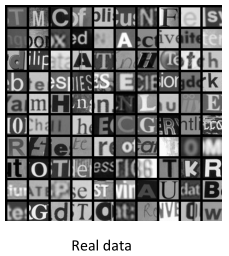• Goal is to take an image patch and have the system recognize the character
• Treat the images as gray-scale (makes it a bit easer)
• How can we amplify this

• Modern computers often have a big font library
• If you go to websites, huge free font libraries
• For more training data, take characters from different fonts, paste these characters again random backgrounds
• After some work, can build a synthetic training set• Random background
• Maybe some blurring/distortion filters
• Takes thought and work to make it look realistic
• If you do a sloppy job this won’t help!
• So unlimited supply of training examples
• This is an example of creating new data from scratch
• Other way is to introduce distortion into existing data

• e.g. take a character and warp it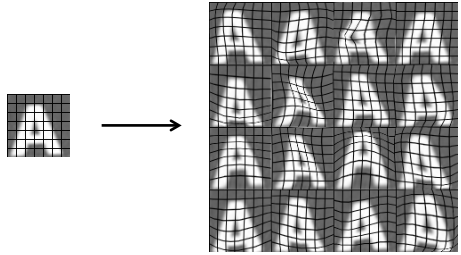• 16 new examples
• Allows you amplify existing training set
• This, again, takes though and insight in terms of deciding how to amplify

• Another example: speech recognition

• Learn from audio clip - what were the words
• Have a labeled training example
• Introduce audio distortions into the examples
• So only took one example
• Created lots of new ones!
• When introducing distortion, they should be reasonable relative to the issues your classifier may encounter
• Getting more data

• Before creating new data, make sure you have a low bias classifier
• Plot learning curve
• If not a low bias classifier increase number of features
• Then create large artificial training set
• Very important question: How much work would it be to get $10\times$ data as we currently have?
• Often the answer is, “Not that hard”
• This is often a huge way to improve an algorithm
• How many minutes/hours does it take to get a certain number of examples
• Say we have 1000 examples
• 10 seconds to label an example
• So we need another 9000 - 90000 seconds
• Comes to a few days (25 hours!)
• Crowd sourcing is also a good way to get data
• Risk or reliability issues
• Cost
• Example: Amazon Mechanical Turk (MTurk)

#### 36.4 Celling Analysis: What Part of the Pipeline to Work on Next

• Through the course repeatedly said one of the most valuable resources is developer time

• Pick the right thing for you and your team to work on
• Avoid spending a lot of time to realize the work was pointless in terms of enhancing performance
• Photo OCR pipeline

• Three modules
• Each one could have a small team on it
• Where should you allocate resources?
• Good to have a single real number as an evaluation metric
• So, character accuracy for this example
• Find that our test set has 72% accuracy
• Ceiling analysis on our pipeline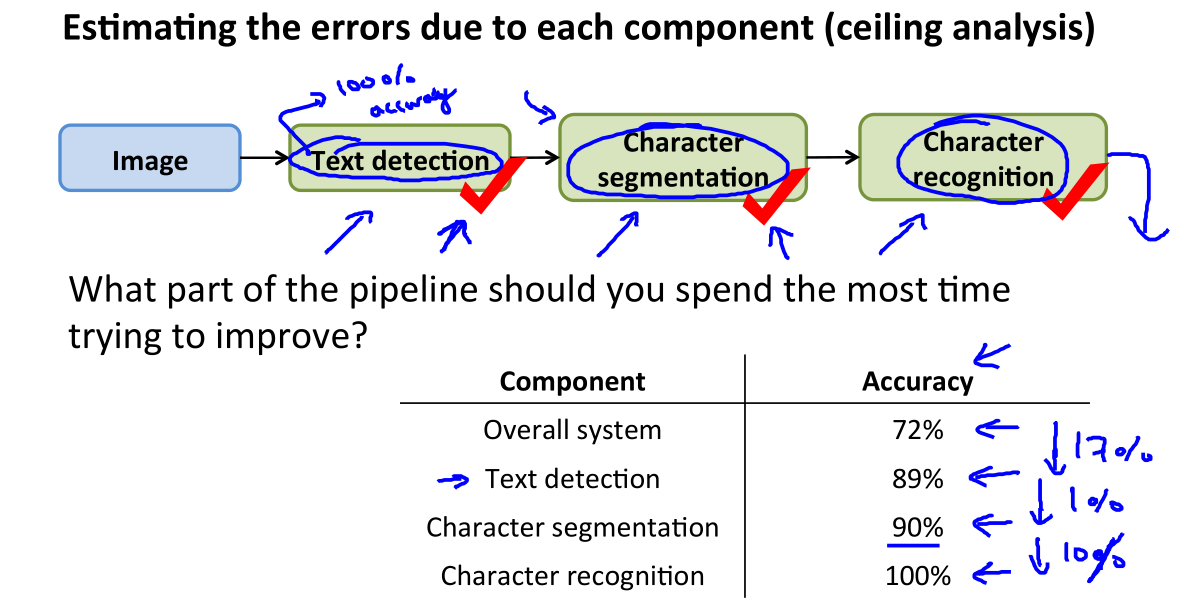• We go to the first module
• Mess around with the test set - manually tell the algorithm where the text is
• Simulate if your text detection system was 100% accurate
• So we’re feeding the character segmentation module with 100% accurate data now
• How does this change the accuracy of the overall system
• Accuracy goes up to 89%
• Next do the same for the character segmentation
• Accuracy goes up to 90% now
• Finally doe the same for character recognition
• Goes up to 100%
• Having done this we can qualitatively show what the upside to improving each module would be
• Perfect text detection improves accuracy by 17%!
• Would bring the biggest gain if we could improve
• Perfect character segmentation would improve it by 1%
• Not worth working on
• Perfect character recognition would improve it by 10%
• Might be worth working on, depends if it looks easy or not
• The “ceiling” is that each module has a ceiling by which making it perfect would improve the system overall
• Another example - face recognition

• This is not how it’s done in practice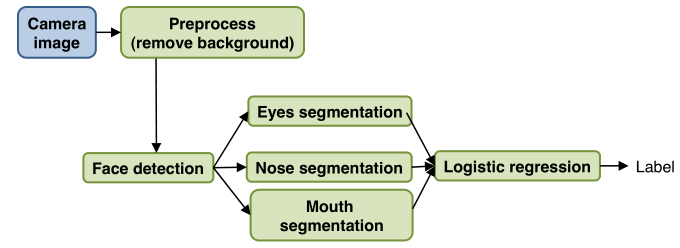• How would you do ceiling analysis for this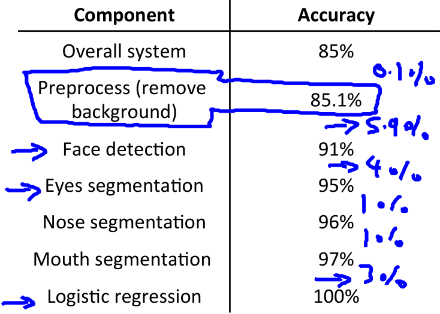## Conclusion

• Supervised Learning
• Linear regression, logistic regression, neural networks, SVMs
• Unsupervised Learning
• K-means, PCA, Anomaly detection
• Special applications/special topics
• Recommender systems, large scale machine learning
• Advice on building a machine learning system
• Bias/variance, regularization; deciding what to work on next: evaluation of learning algorithms, learning curves, error analysis, ceiling analysis
• AND A BIG THANK YOU!

Thistledown